## Introduction

• A pyramid is a three-dimensional solid with a polygonal base and flat triangular (lateral) faces that meet at a point called the apex.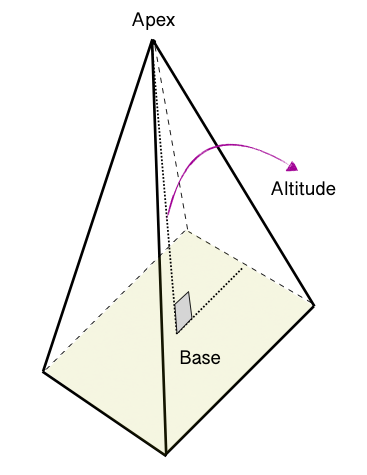• The height or altitude of the pyramid is the line drawn from the apex perpendicular to the base.
• A pyramid is named after the shape of its base. Here are a few different types of pyramids.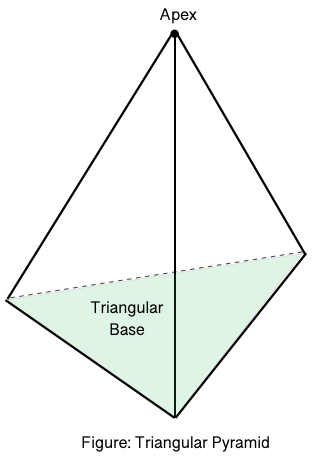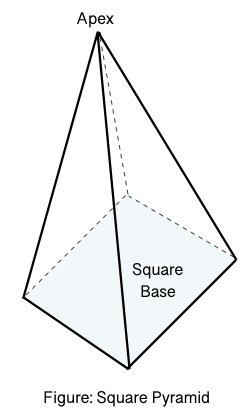• Pyramids can further be classified as regular pyramids and irregular pyramids.
• In a regular pyramid, the base is a regular polygon (all sides are equal), and all the lateral faces are triangles.
• The height of each triangle of a regular pyramid is the slant height of the pyramid.

## Volume of Triangular Pyramids

• The volume of a regular pyramid with the area of the base $\left(B\right)$ and height $\left(h\right)$ can be calculated using the formula mentioned below.

$Volum{e}_{pyramid}=\frac{1}{3}×B×h$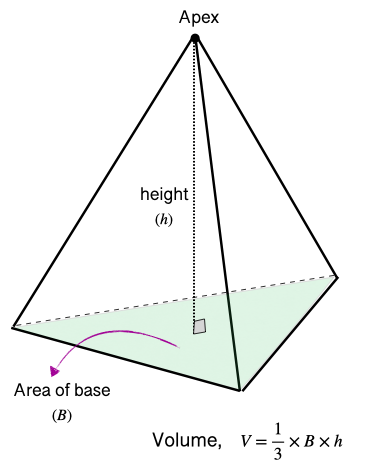## Surface Area of Triangular Pyramids

• A regular triangular pyramid has a triangular base (all sides equal) and three triangular lateral faces.
• The lateral area of a regular pyramid can be found by adding the area of lateral faces.

$LA=\frac{1}{2}×p×s$, where $p=$the perimeter of the base, and $s=$the slant height of the triangular faces.

• The total area of a triangular prism can be found by adding an area of the base and the area of lateral faces.

$TA=LA+B$$=\frac{1}{2}×p×s+B$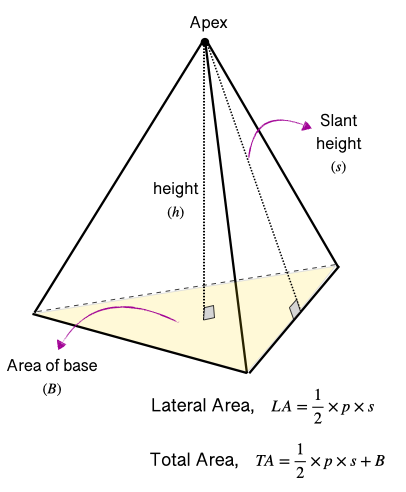## Volume of Square Pyramids

• The volume of a square pyramid with the area of base $\left(B\right)$ and height $\left(h\right)$ can be calculated using the formula mentioned below.

$Volum{e}_{pyramid}=\frac{1}{3}×B×h$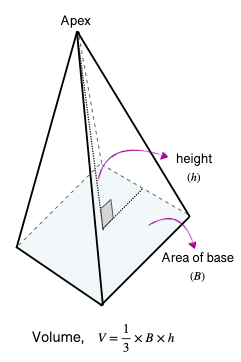## Surface Area of Square Pyramids

• A square pyramid has a square base and four triangular lateral faces.
• The lateral surface area of a square pyramid with a perimeter of the base $\left(p\right)$ and slant height $\left(l\right)$ can be calculated using the formula mentioned below:

$LA=\frac{1}{2}×p×s$

• The total surface area of a square pyramid: $TA=LA+2B$$=\frac{1}{2}×p×s+B$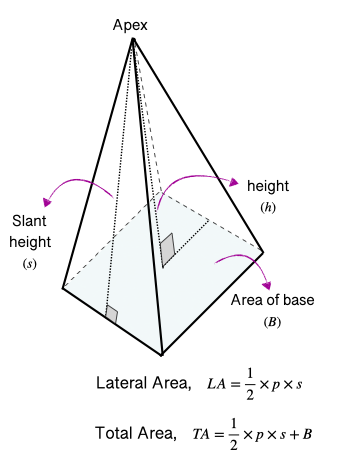## Some Solved Examples

Question 1: A square pyramid has a side length of 6 cm and a height of 10 cm. Find the volume of the square pyramid.

Solution: $Volum{e}_{pyramid}=\frac{1}{3}×B×h$$=\frac{1}{3}×{\left(6\right)}^{2}×10$$=\frac{1}{3}×36×10$$=12×10$

Question 2: The base of a triangular pyramid is an equilateral triangle with a side length of 12 units. If the height of the base triangle is 10 units and the slant height is 11 units, find the total surface area of the pyramid.

Solution: $T{A}_{pyramid}=\frac{1}{2}×p×s+B$$=\frac{1}{2}×\left(3×12\right)×11+\frac{1}{2}×12×10$$=198+60$

## Cheat Sheet

• For any regular pyramid with the area of the base $\left(B\right)$, height $\left(h\right)$, slant height of the base $\left(s\right)$, and perimeter of the base $\left(p\right)$:
• Volume, $Volum{e}_{pyramid}=\frac{1}{3}×B×h$
• Lateral Surface Area, $L{A}_{pyramid}=\frac{1}{2}×p×s$
• Total Surface Area, $T{A}_{pyramid}=L{A}_{pyramid}+B$$=\frac{1}{2}×p×s+B$

## Blunder Areas

• It is essential to know how to find the base's area based on the polygon's shape.
• Sometimes students get confused between a pyramid's slant height and altitude (height).